# Texas Go Math Grade 7 Lesson 6.2 Answer Key Theoretical Probability of Compound Events

Refer to our Texas Go Math Grade 7 Answer Key Pdf to score good marks in the exams. Test yourself by practicing the problems from Texas Go Math Grade 7 Lesson 6.2 Answer Key Theoretical Probability of Compound Events.

## Texas Go Math Grade 7 Lesson 6.2 Answer Key Theoretical Probability of Compound Events

Finding Probability Using a Table

Recall that a compound event consists of two or more simple events. To find the probability of a compound event, you write a ratio of the number of ways the compound event can happen to the total number of equally likely possible outcomes.Jacob rolls two fair number cubes. Find the probability that the numbers he rolls is 8.
STEP 1: Use the table to find the sample space for rolling a particular sum on two number cubes. Each cell is the sum of the first number in that row and column.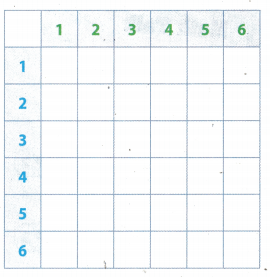STEP 2: How many possible outcomes are in the sample space?
STEP 3: Circle the outcomes that give the sum of 8. ________
STEP 4: How many ways are there to roll a sum of 8? ________
STEP 5: What is the probability of rolling a sum of 8? ________

Reflect

Question 1.
Give an example of an event that is more likely than rolling a sum of 8.
P (rolling a sum of 8) = $$\frac{5}{36}$$ = $$\frac{5}{36}$$
Rolling a sum of 7 is example of an event that is more likely than rolling a sum of 8.
There are six ways to roll a sum of 7.
P (rolling a sum of 7) = $$\frac{6}{36}$$ = $$\frac{1}{6}$$.

Question 2.
Give an example of an event that is less likely than rolling a sum of 8.
5 5
P (rolLing a sum of 8) = $$\frac{5}{36}$$ = $$\frac{5}{36}$$
Rolling a sum of 2 is example of an event that is less likely than rolling a sum of 8.
Only way to roll a sum of 2 is to roll 1 on each cube and probability for that is:
P (rolLing a sum of 2) = $$\frac{1}{36}$$ = $$\frac{1}{36}$$

Example 1

A deli prepares sandwiches with one type of bread (white or wheat), one type of meat (ham, turkey, or chicken), and one type of cheese (cheddar or Swiss). Each combination is equally likely. Find the probability of choosing a sandwich at random and getting turkey and Swiss on wheat bread.
STEP 1: Make a tree diagram to find the sample space for the compound event.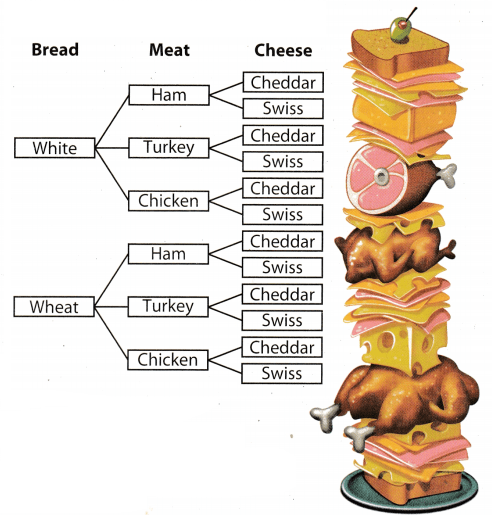STEP 2: Find the number of possible outcomes in the sample space: 12
STEP 3: Find the probability of getting turkey and Swiss on wheat bread at random: $$\frac{1}{12}$$

Use the diagram from Example 1 to find the given probabilities.

Question 3.
P(ham sandwich) _________________________
We have two types of bread for sandwich, and three type of meat, so total number of possible outcomes is 6. Number of favorable outcomes is 2 because we can prepare ham sandwich with one of two types of bread
P (ham sandwich) = $$\frac{2}{6}$$ = $$\frac{1}{3}$$

Question 4.
P(sandwich containing Swiss cheese) ______________
We have two types of bread for sandwich, three types of meat and two types of cheese, so total number of possible outcomes is 12. Number of favorable outcomes is 6 because we can prepare sandwich with Swiss cheese in 6 ways .
P (sandwich containing Swiss cheese) = $$\frac{6}{12}$$ = $$\frac{1}{2}$$

Question 5.
Martha types a 4-digit code into a keypad to unlock her car doors. The code uses the numbers 1 and 0. If the digits are selected at random, what is the probability of getting a code with exactly two 0s?
Let symbols A. B. C, D represent:
A = For first digit in code we have two opportunities: 0 and 1;
B = For second digit in code we have two opportunities: 0 and 1;
C = For third digit in code we have two opportunities: 0 and 1;
D = For forth digit in code we have two opportunities: 0 and 1.
Total number of possible outcomes is:
A ∙ B ∙ C ∙ D = 2 ∙ 2 ∙ 2 ∙ 2 = 16
For favorable outcomes:Drake rolls two fair number cubes. (Explore Activity)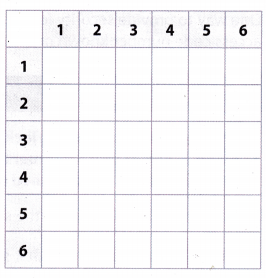Question 1.
Complete the table to find the sample space for rolling a particular product on two number cubes.
Each cell is the product of the first number in that row and column.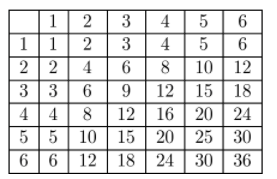Question 2.
What is the probability that the product of the two numbers Drake rolls is a multiple of 4?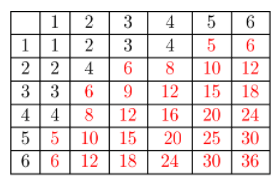Count how many numbers in the table are greater than 4.
The probability that the product of two numbers Drake rolls is a multiple of 4 is number of the product of two numbers that is greater than 4 divided by total number of possible outcomes:
$$\frac{28}{36} \frac{: 4}{: 4} \frac{7}{9}$$
The probability that the product of two numbers Drake rolls is a multiple of 4 is $$\frac{7}{9}$$.

Question 3.
What is the probability that the product of the two numbers Drake rolls is less than 13?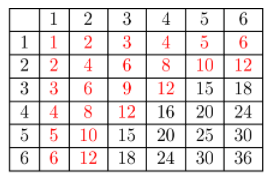Count how many numbers in the table are less than 13.
The probability that the product of two numbers Drake rolls is less than 13 is number of the the product of two numbers that is less than 13 divided by total number of possible outcomes:
$$\frac{23}{36}$$
The probability that the product of two numbers Drake rolls is less than 13 is $$\frac{23}{36}$$.

You flip three coins and want to explore the probabilities of certain events. (Examples 1 and 2)

Question 4.
Complete the tree diagram and make a list to find the sample space.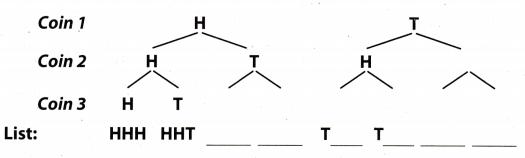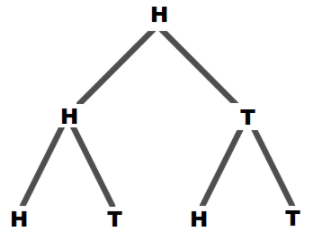List: HHH, HHT, HTH, HTT.List: THH, THT, TTH, TTT.

Question 5.
How many outcomes are in the sample space?
There are 8 outcomes in sample space: HHH, HHT, HTH, HTT, THH, THT, TTH, TTT.

Question 6.
List all the ways to get three tails.
Only way to flip three tails is that a tail falls on each coin, TTT.

Question 7.
Complete the expression to find the probability of getting three tails.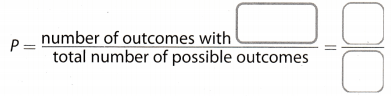The probability of getting three tails when three coins are flipped is _________.The probability of getting three tails when three coins are flipped is $$\frac{1}{8}$$.

Question 8.
What is the probability of getting exactly two heads?
There are ________ way(s) to obtain exactly two heads: HHT, __________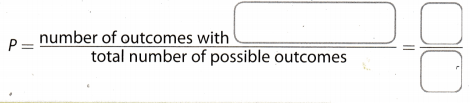There are three ways to obtain exactly two heads. HHT, HTH, THH.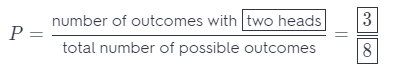P = $$\frac{3}{8}$$

Essential Question Check-In

Question 9.
There are 6 ways a given compound event can occur. What else do you need to know to find the theoretical probability of the event?
Formula for theoretical probability is:
P (event) =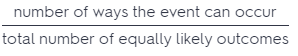We know the number of ways the event can occur (6). therefore, to find a theoretical probability we need information for total number of equally likely outcomes.

In Exercises 10-12, use the following information. Mattias gets dressed in the dark one morning and chooses his clothes at random. He chooses a shirt (green, red, or yellow), a pair of pants (black or blue), and a pair of shoes (checkered or red).

Question 10.
Use the space below to make a tree diagram to find the sample space.The sample space has 12 elements.

Question 11.
What is the probability that Mattias picks an outfit at random that includes red shoes? ___________________________
Total number of possible outcomes is:
3 possibilities for shirt ∙ 2 possibilities for jeans ∙ 2 possibilities for shoes = 3 ∙ 2 ∙ 2 = 12
Number of outfit with red shoes: green-black-red shoes, green-blue-red shoes, red-black-red shoes, red-blue-red shoes, yellow-black-red shoes, yellow-bLue-red shoes = 6
P (outfit with red shoes) =The probability to pick an outfit at random that includes red shoes is $$\frac{1}{2}$$.

Question 12.
What is the probability that no part of Mattias’s outfit is red? _____________________
Total number of possible outcomes is:
3 possibilities for shirt ∙ 2 possibilities for jeans ∙ 2 possibiLities for shoes = 3 ∙ 2 ∙ 2 = 12
Number of outfit without red clothes or shoes: green-black-checkered, green-blue-checkered, yellow-black checkered, yellow-blue-checkered = 4
P (no part of outfit is red) =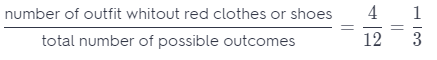The probability that no part of Mattia’s outfit is red is $$\frac{1}{3}$$.

Question 13.
Rhee and Pamela are two of the five members of a band. Every week, the band picks two members at random to play on their own for five minutes. What is the probability that Rhee and Pamela are chosen this week?
Total number of possible outcomes is
5 possibilities to pick one member• 4 possibiLities to pick other member = 5 ∙ 4 = 20
To pick other member we have 4 possibiLities because we already pick one from five for first member so 5 – 1 = 4.
Number of possibilities to pick Pamela and Rhee at the same time is 2.
P (picking Pamela and Rhee) =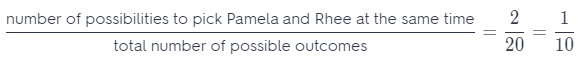The probability that Pamela and Rhee are chosen this week is $$\frac{1}{10}$$

Question 14.
Ben rolls two number cubes. What is the probability that the sum of the numbers he rolls is less than 6?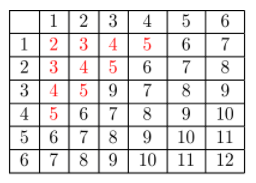Count how many numbers in the table are less than 6.
The probability that the sum of two numbers Ben rolls is less than 6 is number of the sum of two numbers that is less than 6 divided by total number of possible outcomes:
$$\frac{10}{36}$$ = $$\frac{5}{18}$$
The probability that the sum of two numbers Ben rolls is less than 6 is $$\frac{5}{18}$$.

Question 15.
Nhan is getting dressed. He considers two different shirts, three pairs of pants, and three pairs of shoes. He chooses one of each of the articles at random. What is the probability that he will wear his jeans but not his sneakers?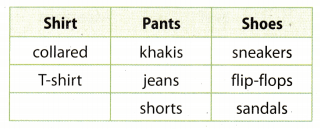Number of combination with jeans but without sneakers: collared shirt, jeans and flip-flops; collared shirt, jeans and sandals; t-shirt, jeans and flip-flops; t-shirt, jeans and sandals.
P (wearing jeans but not sneakers) =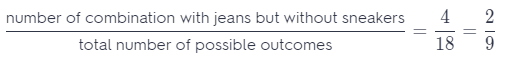The probability that he will wear his jeans but not his sneakers is $$\frac{2}{9}$$.

Question 16.
Communicate Mathematical Ideas A ski resort has 3 chair lifts, each with access to 6 ski trails. Explain how you can find the number of possible outcomes when choosing a chair lift and a ski trail without making a list, a tree diagram, or table.
Each chair has access to 6 ski trails, therefore, number of possible outcomes is 3 ∙ 6 = 18.
Number of possible outcomes is 18.

Question 17.
Explain the Error For breakfast, Sarah can choose eggs, granola or oatmeal as a main course, and orange juice or milk for a drink. Sarah says that the sample space for choosing one of each contains 32 = 9 outcomes. What is her error? Explain.
Sara’s error:She multiply possibilities of her meal which contain milk with eggs, granola and oatmeal and possibilities that contain orange jouce with egg, granola and oatmeal.

Right answer 5: With milk Sarah can choose eggs, granola and oatmeal and that is three possibilities.
With orange juice Sarah can choose eggs, granola and oatmeal, that also three possibilities.
Sample space is: 3 + 3 = 6
The sample space is 6 outcomes.

Question 18.
Represent Real-World Problems A new shoe comes in two colors, black or red, and in sizes from 5 to 12, including half sizes. If a pair of the shoes is chosen at random for a store display, what is the probability it will be red and size 9 or longer?
Total number of possible outcomes: Contain 15 sizes of shoes and two color, so 15 ∙ 2 = 30
P (red and size 9 or larger) =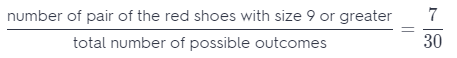The probability to choose red shoes with size 9 or larger is $$\frac{7}{30}$$.

H.O.T. Focus on Higher Order Thinking

Question 19.
Analyze Relationships At a diner, Sondra tells the server, “Give me one item from each column.” Gretchen says, “Give me one main dish and a vegetable.” Who has a greater probability of getting a meal that includes salmon? Explain.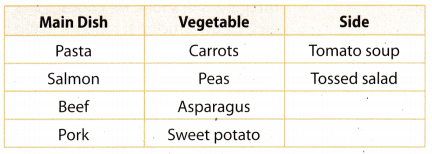Total number of possible outcomes for Sondra: She order main dish, vegetable and side, so 4 possibilities for mean dish 4 possibilities for vegetable ∙ 2 possibiLities for side = 32.

Number of meal with salmon for Sondra: 4 possibilities for vegetable multiply by 2 possibilities for side, so 4 ∙ 2 = 8

The probability of getting a meal that includes salmon for Sondra is:
P (getting a salmon) =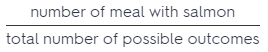= $$\frac{8}{32}$$ = $$\frac{1}{4}$$
Total number of possible outcomes for Gretchen: She order main dish and vegetable, so 4 ∙ 4 ∙ 2 = 16
Number of meal with salmon for Gretchen: 2 possibilities for side, so 1 ∙ 2 = 2

The probability of getting a meaL that includes salmon for Gretchen is:
P (getting a salmon) == $$\frac{2}{16}$$ = $$\frac{1}{8}$$
Sondra has greater possibilities than Gretchen.

Question 20.
The digits 1 through 5 are used for a set of locker codes.
a. Look for a Pattern Suppose the digits cannot repeat. Find the number of possible two-digit codes and three-digit codes. Describe any pattern and use it to predict the number of possible five-digit codes.
Total number of possible outcomes for two-digits code is:
5 possibilities for first digit ∙ 4 possibilities for second digit = 20

Total number of possible outcomes for three-digits code is:
5 possibilities for first digit ∙ 4 possibilities for second digit ∙ 3 possibilities for third digit = 60

Find the number of possible five-digits codes with even number as first digit. Digits do not repeat, and second, third, forth and fifth digit do not depend on number parity.

Total number of possible outcomes for five-digits code S:
2 possibilities for first digit ∙ 4 possibilities for second digit ∙ 3 possibilities for third ∙ 2 possibilities for forth ∙ 1 possibilities for fifth digit = 48

b. Look for a Pattern Repeat part a, but allow digits to repeat.
Total number of possible outcomes for two-digits code is:
5 possibilities for first digit ∙ 5 possibilities for second digit = 25

Total number of possible outcomes for three-digits code is:
5 possibilities for first digit ∙ 5 possibilities for second dig it ∙ 5 possibilities for third digit = 125

Find the number of possible five-digits codes with even number as first digit Digits can be repeated, and second, third, forth and fifth digit do not depend on number parity.

Total number of possible outcomes for five-digits code is:
2 possibilities for first digit ∙ 5 possibilities for second digit ∙ 5 possibilities for third 5 possibilities for forth ∙ 5 possibilities for fifth digit = 1250

c. Justify Reasoning Suppose that a gym plans to issue numbered locker codes by choosing the digits at random. Should the gym use codes in which the digits can repeat or not? Justify your reasoning.